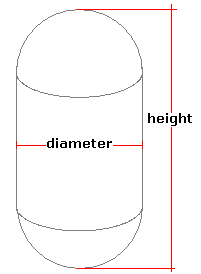Spike's Calculators

# Vertical Cylindrical Tank with Hemispherical HeadsCalculate the volume and capacity of a vertical cylindrical tank with hemispherical heads. The tank has the shape of a capsule.
This calculator uses inches for measurements.

##### Approximate weight of Fuel or Water
```one US gallon of water = 8.34 pounds.
one US gallon of diesel fuel = 7.1 pounds
one US gallon of gas = 6 pounds
```
`Note: measurement used in the calculation is the  diameter, not the radius ! The length measurement is the overall tank length, cylinder plus the two caps.`

### Vertical Cylindrical Tank with Hemispherical Heads

Tank Diameter in
Height of the Tank in
Liquid Weight-Pounds per US Gallon lb/gal US

#### Results:

###### Tank Volume:
In Cubic Inches in³
In Cubic Feet ft³
In Cubic Metres
###### Tank Capacity:
In US Gallons gal US
In Imperial Gallons gal Imperial
In Litres L
###### Liquid Weight and Surface Area
Weight of Liquid lbs
Surface Area in Square Inches in²
In Square Feet ft²
In Square Millimetres mm²
In Square Metres

#### Calculator

1. the diameter of the tank in inches
2. the height of the tank in inches
3. the weight of the liquid in pounds per US gallon

#### Results

1. the volume of the tank in cubic inches
2. the volume in cubic feet
3. the volume in cubic metres
4. the tank capacity in US gallons
5. the capacity in Imperial gallons
6. the capacity in litres
7. full tank liquid weight based on lbs/gal US
8. the surface area of the tank in square inches
9. the surface area in square feet
10. the surface area in square millimetres
11. the surface area in square metres

##### Formula
```V = 𝝅(D/2)²H
where V is the volume
𝝅 = 3.14159265
D the diameter
H the length of the cylinder part of the tank
```
##### Conversions
```one cubic inch (in³) = 0.00432900432900432 gallons (gal) US
one cubic inch (in³) = 0.00360465014990905 gallons (gal) Imperial
one cubic inch (in³) = 0.016387064 litres (L)
```

### Vertical Cylindrical Tank with Hemispherical Heads (Capsule)#### Filled